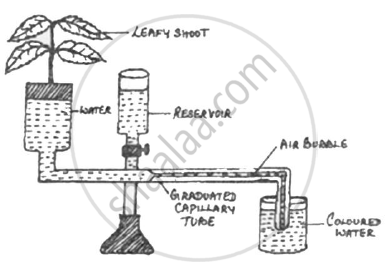Share

# The diagram of an given below demonstrates a particular process in plants. Study the same and answer the questions that follow - ICSE Class 10 - Biology

ConceptRise of Water up to the Xylem

#### Question

The diagram of an given below demonstrates a particular process in plants. Study the same and answer the questions that follow :(i) Name the apparatus.
(ii) Which phenomenon is demonstrated by this apparatus?
(iii) Explain the phenomenon mentioned in (ii) above.
(iv) State two limitations of using this apparatus.
(v) What is the importance of the air bubble in the experiment?
(vi) Name the structures in a plant through which the above process takes place.

#### Solution

(i) The apparatus is called Ganong’s Potometer.
(ii) The phenomenon is called transpiration.
(iii) The evaporative loss of water in the form of water vapour from the aerial parts of plants is known as transpiration.
(iv) Two limitations are

• It is not easy to introduce the air bubble into the capillary.
• The twig may not remain fully alive for a long time.

(v) The air bubble helps measure the rate of transpiration.
(vi) Transpiration occurs through roots.

Is there an error in this question or solution?

#### APPEARS IN

2015-2016 (March) (with solutions)
Question 3.2 | 5.00 marks
Solution The diagram of an given below demonstrates a particular process in plants. Study the same and answer the questions that follow Concept: Rise of Water up to the Xylem.
S